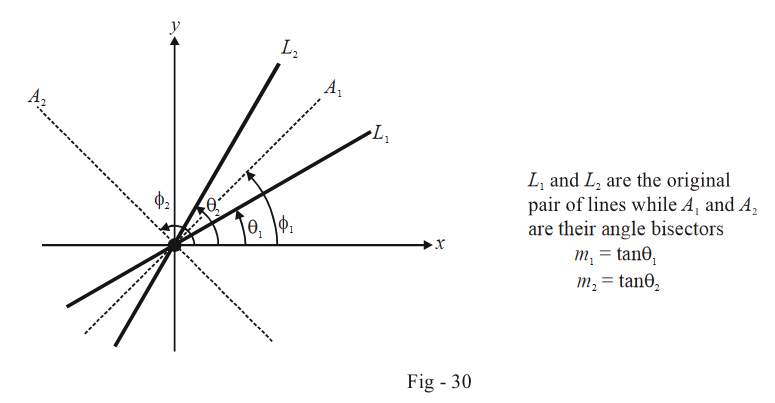# Examples on General Equation of a Pair of Lines Set 1

Example - 21

Prove that the equation $$6{x^2} + 13xy + 6{y^2} + 8x + 7y + 2 = 0$$ represents a pair of straight lines. Find the point of intersection and the angle between these two lines.

Solution: To show that this equation represents a pair of straight lines, we use the determinant condition mentioned earlier:

\begin{align} \left| {\begin{array}{*{20}{c}} a&h&g\\ h&b&f\\ g&f&c \end{array}} \right| & = \left| {\begin{array}{*{20}{c}} 6&{\frac{{13}}{2}}&4\\ {\frac{{13}}{2}}&6&{\frac{7}{2}}\\ 4&{\frac{7}{2}}&2 \end{array}} \right|\\ & = 6\left( {12 - \frac{{49}}{4}} \right) + \frac{{13}}{2}\left( {14 - 13} \right) + 4\left( {\frac{{91}}{4} - 24} \right)\\ & = - \frac{3}{2} + \frac{{13}}{2} - 5\\ & = 0 \end{align}

which confirms the stated assertion.

The angle between these two lines is given by

\begin{align} \tan \theta & = \frac{{2\sqrt {{h^2} - ab} }}{{\left| {a + b} \right|}}\\ & = \frac{5}{{12}}\\ \Rightarrow \qquad \theta & = {\tan ^{ - 1}}\left( {\frac{5}{{12}}} \right) \end{align}

To find the point of intersection, we must factorise the joint equation to obtain the separate equations of the lines. This task can be made easy be observing that since the homogenous part of the given equation is

$6{x^2} + 13xy + 6{y^2} = 0$

which can be factorised as

$(2x + 3y)(3x + 2y) = 0,$

the actual factors of the (original) equation will be of the form

$(2x + 3y + \alpha )\,\,(3x + 2y + \beta ) = 0$

Convince yourself about this argument. $$\alpha {\text{ and }}\beta$$ can easily be evaluated using comparison of coefficients to be 2 and 1 respectively. Thus, the two lines are

\begin{align} {L_1}:2x + 3y + 2 = 0\\ {L_2}:3x + 2y + 1 = 0 \end{align}

so that their point of intersection is, by solving this system of equations, \begin{align}\left( {\frac{1}{5},\,\,\frac{{ - 4}}{5}} \right).\end{align}

Example - 22

Show that the four lines given by the equations

\begin{align}& 3{x^2} + 8xy - 3{y^2} = 0\\ &3{x^2} + 8xy - 3{y^2} + 2x - 4y - 1 = 0 \end{align}

form a square. What is the length of the sides of the square ?

Solution: The first joint equation can be easily factorised to yield

\begin{align} & (3x - y)(x + 3y) = 0\\ \Rightarrow \qquad & 3x - y = 0, \qquad x + 3y = 0 \qquad \qquad ...(1) \end{align}

These are perpendicular lines intersecting at the origin. As described in the previous example, the second joint equation can be factorised as

$(3x - y + \alpha )\,(x + 3y + \beta ) = 0$

where $$\alpha {\text{ and }}\beta$$ can be determined by the comparison of coefficients:

$\alpha = - 1,\;\beta = 1$

The other two sides are thus

\begin{align}3x - y - 1 = 0, \qquad x + 3y + 1 = 0 \qquad \qquad ...(2)\end{align}

From (1) and (2), it should be evident that the four lines form a square. The length $$l$$ of the sides of this square can be evaluated by determining the perpendicular distance between any pair of opposite sides, say $$3x - y = 0{\text{ and }}3x - y - 1 = 0:$$

$l = \frac{{\left| {0 - ( - 1)} \right|}}{{\sqrt {{1^2} + {3^2}} }} = \frac{1}{{\sqrt {10} }}$

Example - 23

Find the joint equation of the angle bisectors of the lines represented by $$a{x^2} + 2hxy + b{y^2} = 0.$$

Solution: Let the slopes of the two lines represented by $$a{x^2} + 2hxy + b{y^2} = 0$$ be $$m_1 \text{ and }m_2,$$ so that $$m_1 \text{ and }m_2$$ are the roots of the quadratic

$b{m^2} + 2hm + a = 0$

Thus,

\begin{align}{m_1} + {m_2} = \frac{{ - 2h}}{b}, \qquad \quad {m_1}{m_2} = \frac{a}{b}\end{align}

It should be obvious that the angle bisectors will also pass through the origin, as shown below:From the figure, observe that

\begin{align} {\phi _1} = \frac{{{\theta _1} + {\theta _2}}}{2}, \qquad {\phi _2} &= \frac{\pi }{2} + \frac{{{\theta _1} + {\theta _2}}}{2}\\ \Rightarrow \qquad 2{\phi _1} = {\theta _1} + {\theta _2}, \qquad 2{\phi _2} &= \pi + {\theta _1} + {\theta _2}\\ \Rightarrow \qquad \qquad \, \tan 2{\phi _1} = \tan 2{\phi _2} & = \tan ({\theta _1} + {\theta _2})\\ & = \frac{{\tan {\theta _1} + \tan {\theta _2}}}{{1 - \tan {\theta _1}\tan {\theta _2}}}\\ & = \frac{{{m_1} + {m_2}}}{{1 - {m_1}{m_2}}}\\ & = \frac{{2h}}{{a - b}} \qquad \qquad \qquad \qquad \qquad ...(1) \end{align}

Now, for any point $$(x,\,y)$$ on the angle bisector $${A_1}({\text{or }}{A_2}),$$

\begin{align} &\tan {\phi _1} = \frac{y}{x} \qquad \qquad \left( {{\text{or }}\tan {\phi _2} = \frac{y}{x}} \right)\\ \Rightarrow \qquad & \tan 2\phi = \frac{{2xy}}{{{x^2} - {y^2}}} \qquad \left( {\text{We have used }}\phi {\text{ to represent both }}{\phi _{\rm{1}}}{\text{ and }}{\phi _2} \right) \end{align}

From this relation and (1), we have

$\boxed{\frac{{{x^2} - {y^2}}}{{a - b}} = \frac{{xy}}{h}}$

This is the joint equation of the angle bisectors; as expected, it is a second degree homogenous equation.

As a corollary, suppose we are required to find the joint equation of the angle bisectors of the lines $$L_1 \text{ and }L_2$$ represented by

$\frac{{{X^2} - {Y^2}}}{{a - b}} = \frac{{XY}}{h}$

We first find the point of intersection of $$L_1 \text{ and }L_2,$$ say $$P(\alpha ,\;\beta ).$$ If we now shift our coordinate system (translation) so that P is the origin (Refer to Appendix - 1), and denote the coordinates in the new system by $$(X,\;Y),$$ we will have the joint equation of the angle bisectors of $$L_1 \text{ and }L_2$$ as

$\frac{{{X^2} - {Y^2}}}{{a - b}} = \frac{{XY}}{h}$

But since $$X \to x - \alpha ,\quad Y \to y - \beta ,$$ the joint equation in the original frame is

$\frac{{{{(x - \alpha )}^2} - {{(y - \beta )}^2}}}{{a - b}} = \frac{{(x - \alpha )(y - \beta )}}{h}$